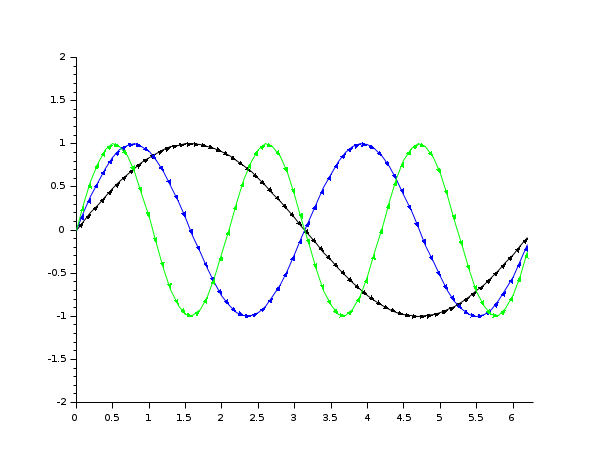Scilab Home page | Wiki | Bug tracker | Forge | Mailing list archives | ATOMS | File exchange
Change language to: Français - Português - 日本語 - Русский
Scilab Help >> Graphics > 2d_plot > plot2d4

# plot2d4

2D plot (arrows style)

### Syntax

```plot2d4([logflag,] x,y,[style,strf,leg,rect,nax])
plot2d4(y)
plot2d4(x,y <,opt_args>)```

### Arguments

args

see `plot2d` for a description of parameters.

### Description

`plot2d4` is the same as `plot2d` but curves are plotted using arrows style. This can be useful when plotting solutions of an ODE in a phase space.

By default, successive plots are superposed. To clear the previous plot, use `clf()`.

Enter the command `plot2d4()` to see a demo.Note that all the modes proposed by `plot2dxx` (xx = 1 to 4) can be enabled using `plot2d` and setting the `polyline_style` option to the corresponding number.

### Examples

Demo: plot2d4();Examples:
```// compare the following with plot2d + .polyline_style=4
clf
subplot(2,1,1)
x = [0:0.1:2*%pi]';
plot2d4(x,[sin(x) sin(2*x) sin(3*x)])

subplot(2,1,2)
plot2d(x,[sin(x) sin(2*x) sin(3*x)])
e = gce();
e.children.polyline_style=4;```• fchamp — direction field of a 2D first order ODE
• plot2d — 2D plot
• plot2d2 — 2D plot (step function)
• plot2d3 — 2D plot (vertical bars)
• subplot — sets the current axes to a chosen cell of the current gridded figure
• clf — Clears and resets a figure or a frame uicontrol
• polyline_properties — description of the Polyline entity properties